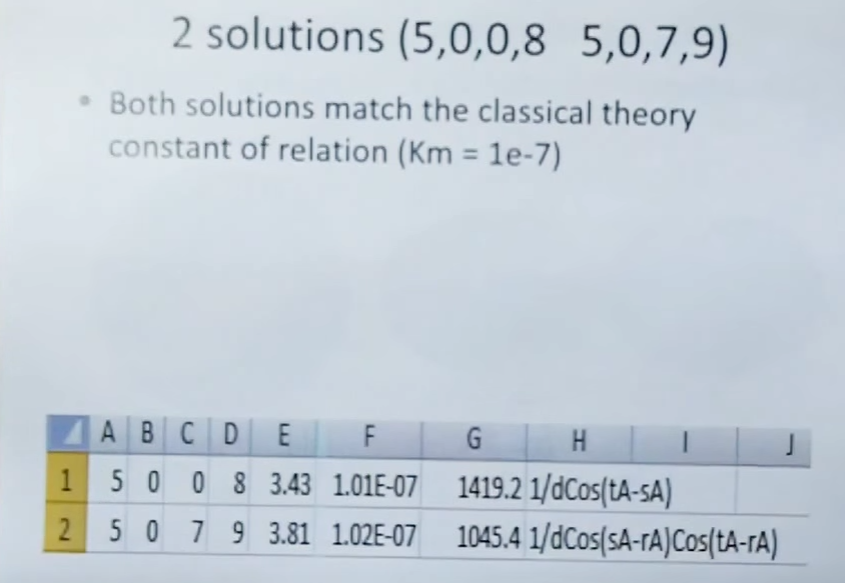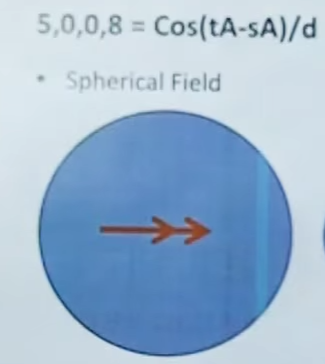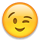# What happens when Solution 5008 cosine is zero

edited April 2020
The solution that best fit Robert Distinti's rhombus experimental data and related analysis was solution number 5008, which was the inverse of the product of (1) the distance (d), and (2) the cosine of the angle of difference of acceleration (tA - sA), between two accelerating charges.

What happens when that cosine goes to zero, because the two charges are accelerating at right angles to each other, as in the rhombus experiment with the largest area, diagonal 25.25 inches, if I recall.

Since cosine of 90 degrees is zero, I would think that this produces a division by zero, indicating that the mutual inductance of two charges accelerating at right angles to each other "blows up" (goes to infinity). That sounds to me like an implausible over-unity result, due to a singularity in the model.

I must be missing something• edited April 2020
That's strange, I will have to rewatch the rhombus videos.• edited April 2020
Ok I think I found the answer in the video emf002r: Rhombus Experiment Data Review

The solution is
`1/dCos(tA-sA)`
You could also read it as
`1/d * Cos(tA-sA)`Later in the same video the equation is written like this:
`Cos(tA-sA) / d`• edited April 2020
Aha - thanks - so the cosine is multiplied, not divided - much better - thanks!
• edited April 2020
So, for the record, another succint, unambiguous way to write Solution 5008 would be: Cos(tA-sA)/d
• edited April 2020
Yes, that's the better way to write the Solution!

And you should not divide it by a zero distance